﻿ Fermat Collocation Method for Solvıng a Class of the First Order Nonlinear Differential Equations

### Fermat Collocation Method for Solvıng a Class of the First Order Nonlinear Differential Equations

Dilek Taştekin, Salih YalçinbaşOPEN ACCESSPEER-REVIEWED

## Fermat Collocation Method for Solvıng a Class of the First Order Nonlinear Differential Equations

Dilek Taştekin1, Salih Yalçinbaş1,1Department of Mathematics Celal Bayar University, Muradiye, Manisa, Turkey

### Abstract

In this study, we present a reliable numerical approximation of the some first order nonlinear ordinary differential equations with the mixed condition by the using a new Fermat collocation method. The solution is obtained in the form of a truncated Fermat series with easily determined components. Also, the method can be used to solve Riccati equation. The numerical results show the effectuality of the method for this type of equations. Comparing the methodology with some known techniques shows that the existing approximation is relatively easy and highly accurate.

1
Prev Next

### Cite this article:

• Taştekin, Dilek, and Salih Yalçinbaş. "Fermat Collocation Method for Solvıng a Class of the First Order Nonlinear Differential Equations." Journal of Mathematical Sciences and Applications 2.1 (2014): 4-9.
• Taştekin, D. , & Yalçinbaş, S. (2014). Fermat Collocation Method for Solvıng a Class of the First Order Nonlinear Differential Equations. Journal of Mathematical Sciences and Applications, 2(1), 4-9.
• Taştekin, Dilek, and Salih Yalçinbaş. "Fermat Collocation Method for Solvıng a Class of the First Order Nonlinear Differential Equations." Journal of Mathematical Sciences and Applications 2, no. 1 (2014): 4-9.

 Import into BibTeX Import into EndNote Import into RefMan Import into RefWorks

### 1. Introduction

Nonlinear ordinary differential equations are frequently used to model a wide class of problems in many areas of scientific fields; chemical reactions, spring-mass systems bending of beams, resistor-capacitor-inductance circuits, pendulums, the motion of a rotating mass around another body and so forth [1, 2]. These equations here also demonstrated their usefulness in ecology, economics, biology, astrophysics and engineering. Thus, methods of solution for these equations are of great importance to engineers and scientists [3, 4].

In this paper, for our aim we consider the first order nonlinear ordinary differential equation of the form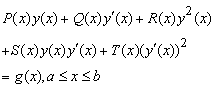(1)

under the mixed conditions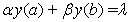(2)

and look for the approximate solution in the form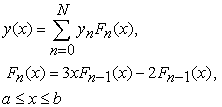(3)

[5, 6]

which is a Fermat polynomial of degree N, where yn(n = 0,1,,N)are the coefficients to be determined. Here P(x), Q(x), R(x), S(x), T(x) and g(x) are the functions defined on axb; the real coefficients α,β and λ are appropriate constants. Note that, if S(x)=T(x)=0 in Eq. (1), it is a Riccati Equation [7, 8, 9].

### 2. Fundamental Matrix Relations

Our aim is to find the matrix form of each term in the nonlinear equation given by Eq. (1). Firstly, we consider the solution y(x) defined by a truncated series (3) and then we can convert to the matrix form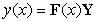(4)

where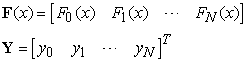If we differentiate expression (4) with respect to x, we obtain(5)

whereOn the other hand, the matrix form of expression y2(x) is obtained as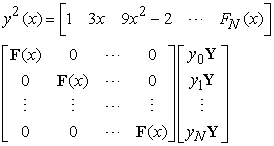or briefly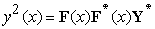(6)

where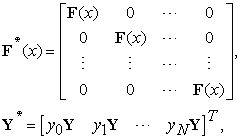By using the expression (4), (5) and (6) we obtain(7)

Following a similar way to (6), we have(8)

where### 3. Matrix Relations Based on Collocation Points

Let us use the collocation points defined by(9)

in order to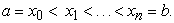By putting the collocation points (9) into Eq. (1), we get the equation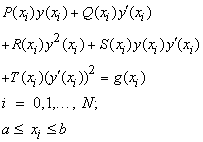(10)

By using the relations (4), (5), (6), (7) and (8); the system (10) can be written in the matrix formor shortly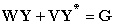(11)

where### 4. Method of Solution

The fundamental matrix equation (11) corresponding to Eq. (1), can be written as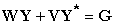or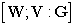(12)

where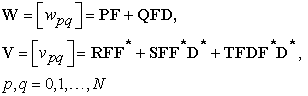We can find the corresponding matrix equation for the condition (2), using the relation (4), as follows:(13)

so thatWe can write the corresponding matrix form (13) for the mixed condition (2) in the augmented matrix form as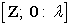(14)

whereTo obtain the approximate solution of Eq. (1) with the mixed condition (2) in the terms of Fermat polynomials, by replacing the row matrix (14) by the last row of the matrix (11), we obtain the required augmented matrix: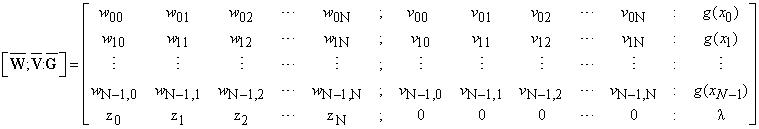or the corresponding matrix equation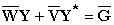(15)

whereThe unknown coefficients set {y0,…,yn} can be determined from the nonlinear system (15). As a result, we can obtain approximate solution in the truncated series form (3).

### 5. Accuracy of Solution

We can check the accuracy of the solution by following procedure [11, 12, 13]: The truncated Fermat series in (3) have to be approximately satisfying Eq. (1); that is, for each x=xi[a,b], i=1,2,and E(xi)10-ki (ki is any positive integer).

If max(10-ki)=10-k (k is any positive integer) is prescribed, then the truncation limit N is increased until the difference E(xi) at each of the points xi becomes smaller than the prescribed 10-k.

### 6. Numerical Examples

In this section, two numerical examples are given to illustrate the accuracy and efficiency of the presented method.

Example 6.1.  Let us first consider the first-order nonlinear differential equation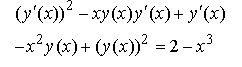(16)

with condition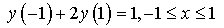and the approximate solution y(x) by the truncated Taylor polynomial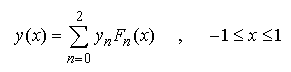whereFor N=2 the collocation points becomeFrom the fundamental matrix equations for the given equation and condition respectively are obtained as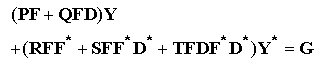andso that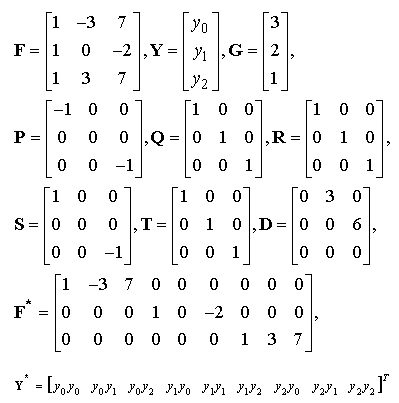The augmented matrix for this fundamental matrix equation is calculated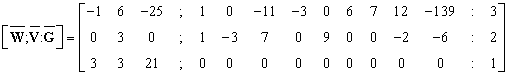From the obtained system, the coefficients y0,y1 and y2 are found as y0=0, y1=1/3 and y2=0.

Hence we have the Fermat polynomial solutionExample 6.2.  Consider the following nonlinear differential equation given by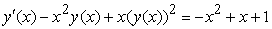(17)

with the initial condition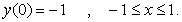So that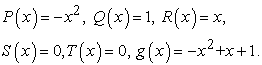For N=2 the collocation points become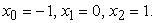From the fundamental matrix equations for the given equation and condition respectively are obtained asand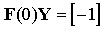so thatThe augmented matrix for this fundamental matrix equation is calculated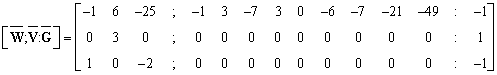From the obtained system, the coefficients y0,y1 and y2 are found as y0=0, y1=1/3 and y2=0.

Hence we have the Fermat polynomial solutionExample 6.3. Consider the following nonlinear differential equation given by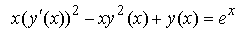(18)

with the initial conditionSo that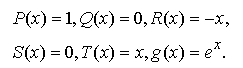The solutions obtained for N=2,5,7 are compared with the exact solution is ex, which are given in Figure 1.We compare the numerical solution and absolute errors for N=2,5,7 in Table 1.

#### Table 1. Comparison of the numerical errors of Example 6.3

Example 6.4. Let us first consider the first-order nonlinear differential equation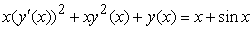(19)

with condition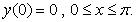Taking N=3,5,7 we obtain the approximate solution of this nonlinear differential equation.

The values of this solution are compared with the exact solution is x+sinx and given errors in Table 2.

### 7. Conclusion

In this study, a new Fermat approximation method for the solution of a class of first order nonlinear differential equations has been presented. The principal advantage of this method is the capability to succeed in the solution up to all term of Fermat expansion. It is seen from Example 6.3 that Fermat collocation method gives well results for the different values N. To obtained numerical results show that accuracy improves when N is increased as shown from Example 6.4. Examples, tables and figures indicate that the present method is convenient, reliable and effective. Also it is important to note that Fermat coefficients of the solution are found very simply by using the computer programs .

### References

  S. L. Ross, Differential Equations, John Wiley and sons, Inc.Newyork, 1974.In article  L. M. Kells, Elementary Differential Equations, Mcgraw-Hill Book Company, Newyork, 1965.In article  Ş. Nas, S. Yalçınbaş and M. Sezer, A Taylor polynomial approach for solving high-order linear Fredholm integro-differential equations, International Journal of Mathematical Education in Science and Technology 31(2), 213-225, 2000.In article CrossRef  S. Yalçınbaş and M. Sezer, The approximate solution of high-order linear Volterra–Fredholm integrodifferential equations in terms of Taylor polynomials, Applied Mathematics and Computation 112, 291-308, 2000.In article CrossRef  A. F. Horadam, University of New England, Armidale, Australia, 1983.In article  L. Carliztz, Restricted Compositions, the Fibonacci Quarterly 14, 3, 254-264, 1976.In article  M. Sezer, A method for the approximate solution of the second order linear differential equations in terms of Taylor polynomials, International Journal of Mathematical Education in Science and Technology 27(6), 821-834, 1996.In article CrossRef  S. Yalçınbaş, Taylor polynomial solutions of nonlinear Volterra-Fredholm integral equations, Applied Mathematics and Computation 127, 195-206, 2002.In article CrossRef  S. Yalçınbaş, K. Erdem, Approximate solutions of nonlinear Volterra integral equation systems, International Journal of Modern Physics B 24(32), 6235-6258, 2010.In article CrossRef  R. A. Adams, Calculus, Addison-Wesley Publihers Limited pp.562, 1990.In article  M. Gulsu, M. Sezer, On the solution of the Riccati Equation by the Taylor Matrix Method, Applied Mathematics and Computation 176 pp. 414-421, 2006.In article CrossRef  K. Erdem, S.Yalçınbaş, Bernoulli Polynomial Approach to High-Order Linear Differential-Difference Equations, AIP Conference Proceedings, 1479, 360-364, 2012.In article CrossRef  S.Yalçınbaş, M.Aynigül, T.Akkaya, Legendre series solutions of Fredholm integral equations, Mathematical and Computational Applications, 15(3), 371-381, 2010.In article  E. Küçükşahin, Taylor polynomial solutions of some first order nonlinear differantial equations, M. Sc. Thesis, Mugla University, 2008.In article  D. Taştekin, S. Yalçınbaş, M. Sezer, Taylor collocation method for solving a class of the first order nonlinear differential equations, Mathematical and Computational Applications, vol.18, no.3, pp.383-391, 2013.In article